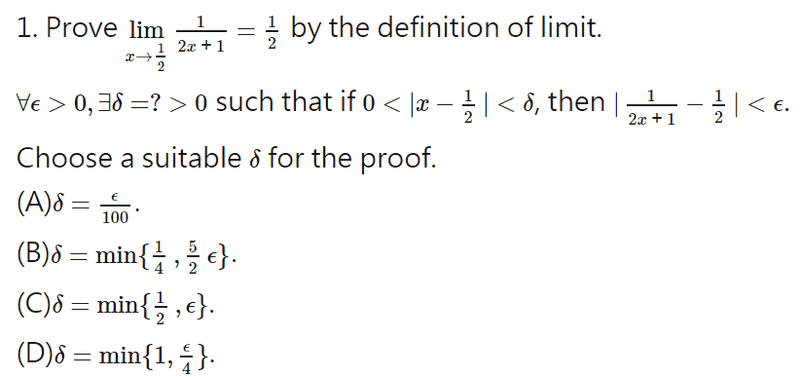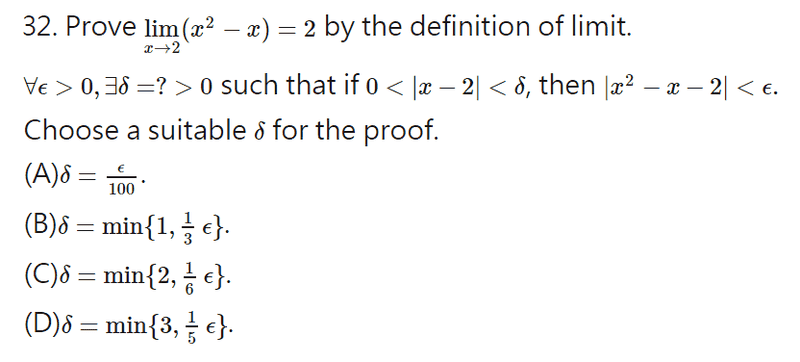# Definition of limit problem

• B
• ChloeYip

#### ChloeYipI have only encountered questions that f(x)-L that can be factorize to get a constant, and delta is epsilon divide that number, as a high school student.
I have no idea how to choose a epsilon for this question.
Thanks.

#### Attachments

if you do some elementary algebraic processing of the two inequalities you will find out that taking ##\delta<=\epsilon## would be enough if we could guarantee that ##(2x+1)>1## or equivalently ##x>0## but in order for that last to happen it must also be ##x-\frac{1}{2}>-\frac{1}{2}## or ##|x-\frac{1}{2}|<\frac{1}{2}##. So delta has to be also ##\delta<=\frac{1}{2}##.

Why δ≤ϵ ? Is it part of the definition of limit? As I know, it only mentioned "for each ϵ>0 there exists δ>0", according to "S. L. Salas, G. J. Etgen, E. Hille,Calculus: One and Several Variables".
For (2x+1)>1 or x>0, are they just an assumption?
Thanks.

Why δ≤ϵ ? Is it part of the definition of limit? As I know, it only mentioned "for each ϵ>0 there exists δ>0", according to "S. L. Salas, G. J. Etgen, E. Hille,Calculus: One and Several Variables".
For (2x+1)>1 or x>0, are they just an assumption?
Thanks.

There are several ways to tackle this problem. But, I think your first difficulty is that you don't really understand the epsilon-delta definition.

In any case, you are given ##\delta## in this question (or, at least, you are given four possibilities). So, why not just try each of them and see where it leads?

(A) Let ##|x - 1/2| < \epsilon/100 \ \dots##

What can you do with that?

Compare |f(x)-L|=|1/(2x+1)| to |x−c|=|x−1/2|, like those in my common practice to elementary exercise?
I really have no idea.

After watching this youtube video: , I tried calculate...
Substitute 1/2-∈ & 1/2+∈ to f(x)=1/(2x+1) → ∂=min{1/[2(1-∈)] or 1/[2(1+∈)]} ?
That's totally different to the given options!
What's wrong with that?

Thanks.

Compare |f(x)-L|=|1/(2x+1)| to |x−c|=|x−1/2|, like those in my common practice to elementary exercise?
I really have no idea.

After watching this youtube video: , I tried calculate...
Substitute 1/2-∈ & 1/2+∈ to f(x)=1/(2x+1) → ∂=min{1/[2(1-∈)] or 1/[2(1+∈)]} ?
That's totally different to the given options!
What's wrong with that?

Thanks.

That may be a solution (I haven't checked). There are lots of possible solutions for how to choose delta (depending on epsilon) in these cases. You have to choose between the four you are given. There will be other ways to tackle this limit.

Let me ask a more general question. Suppose, for another problem, you came up with:

##\delta = \epsilon/2##

Can you see why

##\delta = \epsilon/4##

is also a solution?

Or, do you think those can't both be correct?

Do you mean because they are inequality?
So do you think my steps are correct?

To guess with a more reasonable answer, B or D should be chosen?
I have never learned to put an unknown from denominator to numerator...

Do you mean because they are inequality?
So do you think my steps are correct?

To guess with a more reasonable answer, B or D should be chosen?
I have never learned to put an unknown from denominator to numerator...

I think you should go back to post #4 and follow my suggestion. Try with B) or D) first if you like. In any case, you need to do some algebra.

Is it B?

|[1/(2x+1)]-1/2|=1/2<ϵ → ϵ can be ≥ 1/4 as the only answer.
Am I right?

And how to get 5/2ϵ?

Thanks.

Is it B?

|[1/(2x+1)]-1/2|=1/2<ϵ → ϵ can be ≥ 1/4 as the only answer.
Am I right?

And how to get 5/2ϵ?

Thanks.

Other than Q1,
May I ask one more question(Q32)?I can't even find a constant in Q32.

The following are my steps in Q32:
lx^(2)-x-xl=l(x-2)(x+1)l<ϵ
Let M=x+1,Mδ<ϵ → δ<ϵ/M
l(x-2)l<1 → -1<x-2<1 → 1<x<3
2<x+1<4 → M=4 → δ<ϵ/4 → NO THIS OPTION!
What's wrong with that?

Thanks.

Other than Q1,
May I ask one more question(Q32)?
View attachment 102111
I can't even find a constant in Q32.

The following are my steps in Q32:
lx^(2)-x-xl=l(x-2)(x+1)l<ϵ
Let M=x+1,Mδ<ϵ → δ<ϵ/M
l(x-2)l<1 → -1<x-2<1 → 1<x<3
2<x+1<4 → M=4 → δ<ϵ/4 → NO THIS OPTION!
What's wrong with that?

Thanks.
So B) doesn't work , because according to B) you take ##\delta\leq\frac{\epsilon}{3}## and this choice doesn't necessarily satisfies ##\delta<\frac{\epsilon}{4}##

Check C) which I believe is the right answer. If all go well you 'll find that delta has to satisfy ##\delta<\frac{\epsilon}{5}##. But C) tells you that you take ##\delta\leq\frac{\epsilon}{6}## which for sure satisfies ##\delta<\frac{\epsilon}{5}##.

Is it B?

|[1/(2x+1)]-1/2|=1/2<ϵ → ϵ can be ≥ 1/4 as the only answer.
Am I right?

And how to get 5/2ϵ?

Thanks.

Well, unfortunately, as I said before, your fundamental problem is that you don't really understand what you're trying to do with these epsilons and deltas. You've been given some strong hints and suggestions in this thread, but you don't seem able to follow them up.

My only suggestion is to try to understand what that definition really means. Questions like these should help you, but at the moment you seem to be struggling in the dark and it's difficult to help.

I agree my fundamental problem is that I don't really understand what I'm trying to do with these epsilons and deltas, but I don't really know what can I do with that. I just learn those things online, with only telling me to substitute numbers into the definition written. Are there any suggestions for what can I do other than asking question here? Thanks.

I agree my fundamental problem is that I don't really understand what I'm trying to do with these epsilons and deltas, but I don't really know what can I do with that. I just learn those things online, with only telling me to substitute numbers into the definition written. Are there any suggestions for what can I do other than asking question here? Thanks.

It's not easy, that's for sure. Almost everyone takes some time to get to grips with this. Also, there is no formulaic approach to these questions. There's no technique, like there might be for solving a certain differential equation, for example, that you can simply learn. In fact, to do real analysis you need to be good with a whole range of algebraic techniques. You will start to see common themes and ideas, but you need to be generally good with algebra and numbers.

First, let me try to describe the idea behind epsilon-delta limits. If we take a point, ##x_0## and we have a function ##f(x)## what we are trying to do is to show that as ##x \rightarrow x_0## we have ##f(x) \rightarrow L##. That means that as ##x## gets close to ##x_0## we must have ##f(x)## getting close to ##L## (and staying close to ##L##).

The epsilon-delta definition you quoted in post #1 captures this idea formally and rigorously. At the centre of the definition is an implication: a certain condition on ##x## must imply a certain condition on ##f(x)##. The key points are:

You must be able to prove the implication for any ##\epsilon##.

The ##\delta## you choose in each case depends on ##\epsilon##.

You must show that ##0 < |x - x_0| < \delta## implies ##|f(x) - L| < \epsilon##.

So, in general, you would start by saying:

1) Let ##\epsilon > 0##.

2) Choose ##\delta = \dots## (this is the first tricky bit: you have to find your delta for each epsilon).

3) If ##0 < |x - x_0| < \delta## then ##\dots## (this is the second tricky bit. By algebraic, logic, and any means at your disposal, you must have a rock-solid series of logical steps that leads to ##\dots \ |f(x) - L| < \epsilon##

This is as far as you can go in general. In step 3) almost any algebraic trick you could think of might be needed. For step 3) you really need to think on your feet.

In these questions, they have given you some options for ##\delta##. So, you need to focus on step 3).

The first thing I would do is to work on the expression ##|f(x) - L|##. You need to find how this expression depends on ##|x-x_0|##. Let's take your first question as an example:

##|\frac{1}{2x+1} - \frac{1}{2}| = |\frac{2 - (2x+1)}{2(2x+1)}| = |\frac{1-2x}{2(2x+1)}| = |\frac{2x - 1}{2(2x+1)}| = |\frac{x - 1/2}{2x+1}|##

Do you see what I did in those last two steps? We're now getting somewhere. But, we need to do something about the ##x## in the denominator. Any ideas?

•Delta2
rationalize them?

I now know how to get δ=0.5
the following are the steps:
If δ<1, then lx-0.5l<δ<1 ⇒ -1<x-0.5<1 ⇒-0.5<x-0.5<1.5 ⇒ ϵ = 0.5
Am I right? Thanks

In these questions, they have given you some options for ##\delta##. So, you need to focus on step 3).
In fact, it is a multiple choice question and I think we need to focus on answering it first (i.e. to find δ), then learn ways to solve it...
And as I know, finding delta is the essential to learn to solve it. Hope you may help me learn it first because I still don't understand how to get it done.
Thank you very much for helping me~

I now know how to get δ=0.5
the following are the steps:
If δ<1, then lx-0.5l<δ<1 ⇒ -1<x-0.5<1 ⇒-0.5<x-0.5<1.5 ⇒ ϵ = 0.5
Am I right? Thanks
This is not correct. To get back on track you need to go back to the last expression in post #14 and deal with the denominator.

hint: What happens if ##x > 0##? Would that help?

I'll be offline for a bit, but hopefully someone else can help.